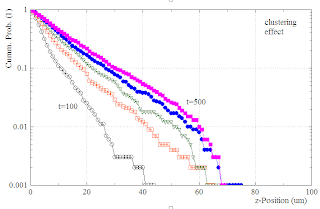## Saturday, August 8, 2009

###  Cell-Invasion : The clustering effect

Last week I had a nice collaboration with Sebastian Probst. He had a very interesting idea:

Assume the agents have a tendency to form and stabilize clusters: Each time they happen to find another closeby agent on their random walk, they drastically reduce their diffusion constant. They won't stop moving entirely, but let's assume they slow down whenever they are in happy company. How would that clustering effect change the invasion process ?

In order to model this idea, we first start again with the simple diffusion simulation from the last post . In regular time intervalls of 100 units, we count how many agents are found within each z-stripe, resulting in a histogram N(z). From this histogram we compute a cumulative probability distribution P_cum(z), which gives the probability that an agent is found in depth z of the material or deeper. In the following, we call this cumulative distributions the invasion profiles.

One finds for the simple diffusion
P_cum(z)-curves which have a negative curvature and approximately Gaussian shapes with a variance that grows with time. Note that this is a semi-log plot, so true Gaussians would show up as downward parabolas.Now we leave everything as before, except that the clustering effect is additionally implemented. For this purpose, we count the number of direct neighbors of each agent. If this number is larger than zero for certain agents, we reduce the hopping rates of these specific agents by a factor of 10 during the next MC cycle. Again we plot the invasion profiles:The result is remarkable:

As a consequence of the clustering effect, the profiles are changed from about Gaussian to almost exponential shape (showing up as linear lines in the semi-log plot).

This offers a completely new, simple, and testable mechanism for explaining the mostly exponential invasion profiles observed in the experiments. It does not require any explicit assumptions about the diffusion of chemical signalling molecules. The only parameters are the maximum diffusion constant of the agents, their detection range for sensing closeby fellows, and the reduced diffusion constant in the clustering mode.

Future tests:

• The initial density of the cells in the monolayer should drastically affect the invasion process. A small density will reduce the probability of cluster formation and thus drive the system back to the normal, Gaussian diffusion of the agents.